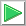# Shape Classification

 Tip It is possible to train an ANN using a sequence of shapes. Each shape can be represented as a sequence of numbers. These numbers can be applied directly to an ANN. However, it is always recommended to process the shape data before using it for training. This processing can be simple mapping operations, or it can be very complicated to highlight those properties that are important for the experiment (see figure below). For instance, the data can be in the time domain or in the frequency domain.Problem 1 Create a New Project called CardiRose (You must select: Multi-layer Network and Classification in the New Project Dialog) to classify two shapes. Edit the BuildTrainSet.lab to build an appropriate training set for the classification of two shapes: the Cardioid and the Rose. The input training set must include the shapes in different phases uniformly distributed : 1000 Cardioid shapes and 1000 Rose shapes. The classifier must be able to tolerate inputs contaminated with 10% of noise. The figure below shows the training process using perfect shapes and noisy shapes. Be sure to scale both shapes to have the same amplitude.Solution 1 After editing the file,Runclick the button to execute the code. If you do not have any errors, the training set will be generated and displayed on the variable list and the file list. Click on the file to see its contents. The first 1000 rows of the training set are the Cardioid shape; the second 1000 rows of the training set are the Rose shape. The training set target has two columns, the first column is for class 1 (Cardioid) and the second column is for class 2 (Rose).

 CardiRose\BuildTrainSet.lab int numCases = 1000; int numInputs = 64; double deltaInput = 6.28/numInputs; double delta = 6.28/(numCases-1); int i; int j; double phase = 0.0; Matrix noise; //________________________________ Class 1: cardioid Matrix inputCardioid; inputCardioid.Create(numCases, numInputs); Matrix targetCardioid; targetCardioid.Create(numCases, 2); double theta = 0.0; for(i=0; iProblem 2 Edit the BuilValidSet.lab file to build an appropriate validation set for problem 1 using random phase for the shapes. Include 175 Cardioids and 150 Roses.

 Solution 2 After editing the file,Run click the button to execute the code. If you do not have any errors, the validation set will be generated and displayed on the variable list and the file list. Use the scroll bar at the top to visualize each validation case. Review the output of the network to verify that the classification is performed correctly.

 CardiRose\BuildValidSet.lab int numCasesCardioid = 150; int numCasesRose = 175; int numInputs = 64; double deltaInput = 6.28/numInputs; int i; int j; double phase = 0.0; Matrix noise; //________________________________ Class 1: cardioid Matrix inputCardioid; inputCardioid.Create(numCasesCardioid , numInputs); Matrix targetCardioid; targetCardioid.Create(numCasesCardioid , 2); double theta = 0.0; for(i=0; i

 Problem 3 Edit the Train.lab file to design and train an ANN for the classification of the shapes.

 Solution 3 After editing the file,Run click the button to execute the code. If you do not have any errors, the ANN will be trained. Wait until the code execution stops. Double click the network in the variable list to see the network. Then, select the trainSetInput.csv file and, the trainSetTarget.csv file. You will be able to see the network behavior in real time by moving the scrollbar at the right.

 CardiRose\Train.lab //_________________________ Network Setup LayerNet net; net.Create(64, 12, 0, 2); int i = 0; //____________________________ Input Scaling for(i = 0; i<64; i++) {      net.SetInScaler(i, 0, 2); // Input values are from 0 to 2 } //____________________________ Output Scaling for(i = 0; i<2; i++) {      net.SetOutScaler(i, 0.0, 1.0); // Output values are from 0 to 1 } //________________________ Load and set the training set Matrix trainSetInput; trainSetInput.Load(); Matrix trainSetTarget; trainSetTarget.Load(); net.SetTrainSet(trainSetInput, trainSetTarget, false); //________________________ Train net.TrainSimAnneal(100, 100, 15, 0.01, false, 4, 1.0e-12); net.TrainConjGrad(2500,1.0e-12); //_____________________________ Save the trained network net.Save();

 Tip In the figure below the input of the network is a Cardioid, observe that the value in output 1 is one while the value in output 2 in zero as expected.Problem 4 Edit the CheckTraining.lab file to check the training: (a) Compute the confusion matrix using the training set. (b) Plot the error for each network output. (c) Save the confusion matrix as a vector image (trainConf.emf).

 Solution 4 (a) After editing the file,Run click the button to execute the code. If you do not have any errors, the number of errors will be displayed in the variable list. Click the variable called trainConf to view the confusion matrix for the training set, it should have zero errors as shown below.

 CardiRose\CheckTraining.lab //_________________________________________ Load the Training Set Matrix trainSetInput; trainSetInput.Load(); Matrix trainSetTarget; trainSetTarget.Load(); //_________________________________________ Load the ANN LayerNet net; net.Load(); //_________________________________________ Run Matrix output = net.Run(trainSetInput); //_________________________________________ Compute the Confusion Matrix Matrix trainConf = ConfusionMatrix(output, trainSetTarget, 0.5); trainConf.Save(); //_________________________________________ Compute the Number of Errors int numErrors = toint(trainConf.GetSum()) - toint(trainConf.GetDiagonalSum());Problem 5 Edit the Validation.lab file to perform the validation of the ANN. (a) Compute the confusion matrix using the validation set. (b) Plot the error for each network output. (c) Save the confusion matrix as a vector image (validConf.emf).

 Solution 5 (a) After editing the file,Run click the button to execute the code. You will be asked to save the file, set the file name to Validation.lab. If you do not have any errors, the number of errors will be displayed in the variable list. Click the variable called validConf to view the confusion matrix for the validation set.

 CardiRose\Validation.lab //_________________________________________ Load the validation set Matrix validSetInput; validSetInput.Load(); Matrix validSetTarget; validSetTarget.Load(); //_________________________________________ Load the ANN LayerNet net; net.Load(); //_________________________________________ Run Matrix output = net.Run(validSetInput); //_________________________________________ Compute the confusion matrix Matrix validConf = ConfusionMatrix(output, validSetTarget, 0.5); validConf.Save(); //_________________________________________ Compute the Number of Errors int numErrors = toint(validConf.GetSum()) - toint(validConf.GetDiagonalSum());Problem 6 Generate a report in Microsoft Word. Write some conclusions in the report focusing on the problems that were faced during the simulation and how these problems were or could be solved.